Basic Mathematics

# Triangles - Calculating Area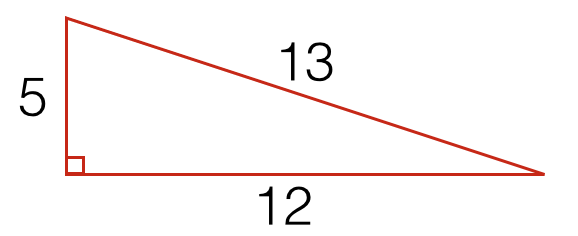What is the area of the right triangle above with side lengths $5, 12,$ and $13$?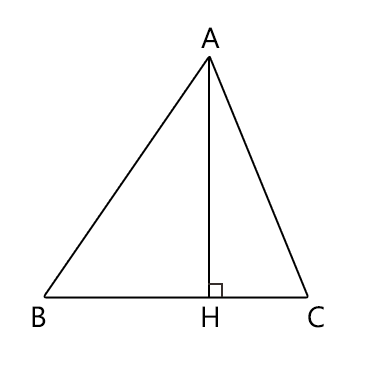In triangle $ABC$ above, the lengths of some line segments are as follows: $\begin{array}{c}&BH=3, &CH=2, &AH=4. \end{array}$ What is the ratio of the area of $\triangle ABH$ to the area of $\triangle ACH?$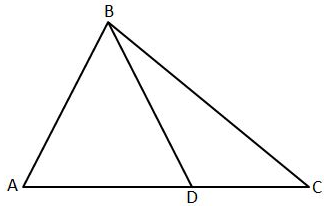In the above diagram, the area of $\triangle ABD$ is $25$ and the area of $\triangle BCD$ is $16.$ What is the ratio of the length of $\overline{AD}$ to the length of $\overline{DC}?$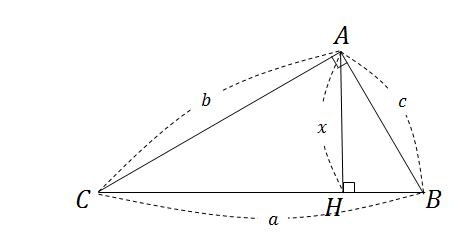In the above diagram, $a=10, b=8, c=6,$ and $\angle CAB$ and $\angle AHB$ are right angles. Find the value of $x.$

Hint: Find two different ways to represent the area (one using the legs and one using the hypotenuse) and set them equal.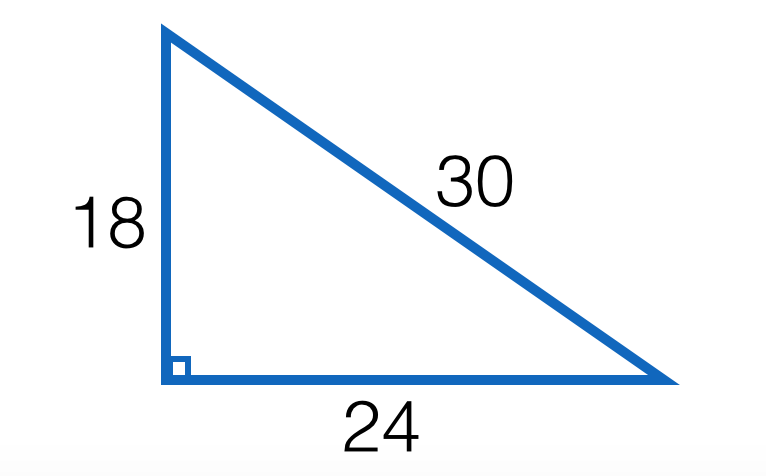What is the area of a triangle with side lengths 18, 24, and 30?

×